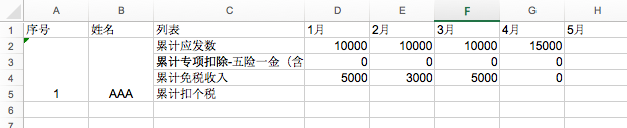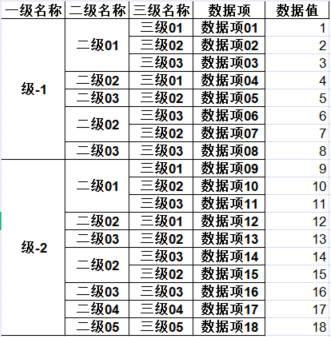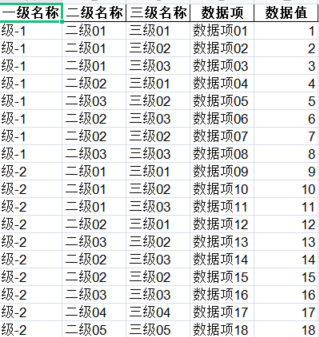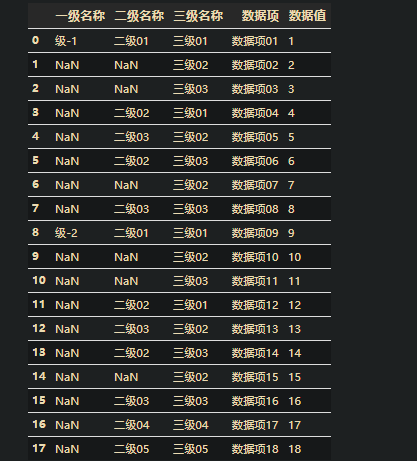• 再次编辑中，这次是在使用删除列的时候发现，合并单元格会出现漏删除情况，才想到用拆分单元格，没想到unmerge_cells（），worksheet.merged_cells返回的合并单元格对象居然不能迭代，函数参数也变了，居然可以直接...
再次编辑中，这次是在使用删除列的时候发现，合并单元格会出现漏删除情况，才想到用拆分单元格，没想到unmerge_cells（），worksheet.merged_cells返回的合并单元格对象居然不能迭代，函数参数也变了，居然可以直接上参数；；openpyxl=Version: 2.5.9；列 ： worksheet.delete_cols(2, 1) 表示第二列开始，删除一列  ，行 worksheet.delete_rows(2, 1)worksheet.unmerge_cells(start_row=1, start_column=7, end_row=2, end_column=7)表示第一行开始，第二行结束， 低7列开始第七列结束， 就是把G1:G2合并的单元格给拆分了，下面的是合并单元格就不多说了worksheet.merge_cells(start_row=1, start_column=2, end_row=2, end_column=2)使用 openpyxl 库拆分已经合并的单元格；主要是使用了：worksheet.merged_cells获取已经合并单元格的信息；再使用worksheet.unmerge_cells（）拆分单元格；importopenpyxlworkbook= openpyxl.load_workbook(path)  #加载已经存在的excel#workbook = openpyxl.Workbook(path)name_list =workbook.sheetnames#worksheet = workbook.get_sheet_by_name(name_list)  #最新版本已经不能使用这种方法worksheet =workbook[name_list]m_list= worksheet.merged_cells  #合并单元格的位置信息，可迭代对象（单个是一个"openpyxl.worksheet.cell_range.CellRange"对象），print后就是excel坐标信息cr =[]for m_area inm_list:#合并单元格的起始行坐标、终止行坐标。。。。，r1, r2, c1, c2 =m_area.min_row, m_area.max_row, m_area.min_col, m_area.max_col#纵向合并单元格的位置信息提取出if r2 - r1 >0:cr.append((r1, r2, c1, c2))print("符合条件%s" %str(m_area))#这里注意需要把合并单元格的信息提取出再拆分for r incr:worksheet.unmerge_cells(start_row=r, end_row=r,start_column=r, end_column=r)workbook.save(path)再使用 worksheet.unmerge_cells（）输入参数的时候，若和 worksheet.merged_cells的结果放到同一个循环；经常出现有些单元格拆分没有完成（实际上可以多次运行就可以完成了），我估计是再拆分单元格的时候有数据动态的改变了，导致（再执行m_area.min_row时）m_list中的"openpyxl.worksheet.cell_range.CellRange"对象动态获取值时发生了改变；只好把位置信息提取出，再调用；后面我测试过m_list对象deepcopy一份就可以了；from copy importdeepcopyl=deepcopy(m_list)for m_area inl:#合并单元格的起始行坐标、终止行坐标。。。。，r1, r2, c1, c2 =m_area.min_row, m_area.max_row, m_area.min_col, m_area.max_colif r2 - r1 >0:worksheet.unmerge_cells(start_row=r1, end_row=r2,start_column=c1, end_column=c2)
展开全文• 利用Python实现Excel合并单元格 最近在工作中需要批量处理一些Excel文件，为了更直观的展示数据，需要对Excel中的单元格进行合并处理。因为一直使用Pandas实现Excel读写操作，而Pandas无法将单元格进行合并，利用...
利用Python实现Excel合并单元格
最近在工作中需要批量处理一些Excel文件，为了更直观的展示数据，需要对Excel中的单元格进行合并处理。因为一直使用Pandas实现Excel读写操作，而Pandas无法将单元格进行合并，利用Python的xlsxwriter模块可以实现Excel合并单元格。帖子 python之DataFrame写excel合并单元格 中提供了一种方法，但在使用中会出现一些问题，比如无法处理字符串等类型数据。通过修改方法，定义了一个excel_merge_cells函数，基本可以满足日常利用Python实现Excel合并单元格的需求。
原帖方法
在工作中经常遇到需要将数据输出到excel，且需要对其中一些单元格进行合并，比如如下表表格，需要根据A列的值，合并B、C列的对应单元格1、定义一个MY_DataFrame类，继承DataFrame类，这样能很好的利用pandas的很多特性，而不用自己重新组织数据结构。
2、定义一个my_mergewr_excel方法，参数分别为：输出excel的路径、用于判断是否需要合并的key_cols列表、用于指明哪些列上的单元格需要被合并的列表
3、将MY_DataFrame封装为一个My_Module模块，以备重用。
合并的算法如下：
1、根据给定参数的【关键列】，进行分组计数和排序，添加CN和RN两个辅助列
2、判断CN大于1的，该分组需要合并，否则该分组（行）无需合并（CN=1说明这个分组数据行是唯一的，无需合并）
3、对应需要合并的分组，判断当前列是不是在给定参数【合并列】中，是则用合并写excel单元格，否则就是普通的写excel单元格。
4、在需要合并的列中，如果对于的RN=1则调用merge_range，一次性写想下写CN个单元格，如果RN>1则跳过该单元格，因为在RN=1的时候，已经合并写了该单元格，若再重复调用erge_range，打开excel文档时会报错。
用图解释如下：具体代码如下：

# -*- coding: utf-8 -*-
"""
Created on 20170301
@author: ARK-Z
"""
import xlsxwriter
import pandas as pd

class My_DataFrame(pd.DataFrame):
def __init__(self, data=None, index=None, columns=None, dtype=None, copy=False):
pd.DataFrame.__init__(self, data, index, columns, dtype, copy)

def my_mergewr_excel(self,path,key_cols=[],merge_cols=[]):
# sheet_name='Sheet1', na_rep='', float_format=None, columns=None, header=True, index=True, index_label=None, startrow=0, startcol=0, engine=None, merge_cells=True, encoding=None, inf_rep='inf', verbose=True):
self_copy=My_DataFrame(self,copy=True)
line_cn=self_copy.index.size
cols=list(self_copy.columns.values)
if all([v in cols for i,v in enumerate(key_cols)])==False:     #校验key_cols中各元素 是否都包含与对象的列
print("key_cols is not completely include object's columns")
return False
if all([v in cols for i,v in enumerate(merge_cols)])==False:  #校验merge_cols中各元素 是否都包含与对象的列
print("merge_cols is not completely include object's columns")
return False

wb2007 = xlsxwriter.Workbook(path)
worksheet2007 = wb2007.add_worksheet()
format_top = wb2007.add_format({'border':1,'bold':True,'text_wrap':True})
format_other = wb2007.add_format({'border':1,'valign':'vcenter'})
for i,value in enumerate(cols):  #写表头
#print(value)
worksheet2007.write(0,i,value,format_top)

#merge_cols=['B','A','C']
#key_cols=['A','B']
if key_cols ==[]:   #如果key_cols 参数不传值，则无需合并
self_copy['RN']=1
self_copy['CN']=1
else:
self_copy['RN']=self_copy.groupby(key_cols,as_index=False).rank(method='first').ix[:,0] #以key_cols作为是否合并的依据
self_copy['CN']=self_copy.groupby(key_cols,as_index=False).rank(method='max').ix[:,0]
#print(self)
for i in range(line_cn):
if self_copy.ix[i,'CN']>1:
#print('该行有需要合并的单元格')
for j,col in enumerate(cols):
#print(self_copy.ix[i,col])
if col in (merge_cols):   #哪些列需要合并
if self_copy.ix[i,'RN']==1:  #合并写第一个单元格，下一个第一个将不再写
worksheet2007.merge_range(i+1,j,i+int(self_copy.ix[i,'CN']),j, self_copy.ix[i,col],format_other) ##合并单元格，根据LINE_SET判断需要合并几个
#worksheet2007.write(i+1,j,df.ix[i,col])
else:
pass
#worksheet2007.write(i+1,j,df.ix[i,j])
else:
worksheet2007.write(i+1,j,self_copy.ix[i,col],format_other)
#print(',')
else:
#print('该行无需要合并的单元格')
for j,col in enumerate(cols):
#print(df.ix[i,col])
worksheet2007.write(i+1,j,self_copy.ix[i,col],format_other)

wb2007.close()
self_copy.drop('CN', axis=1)
self_copy.drop('RN', axis=1)


调用代码：


import My_Module

DF=My_DataFrame({'A':[1,2,2,2,3,3],'B':[1,1,1,1,1,1],'C':[1,1,1,1,1,1],'D':[1,1,1,1,1,1]})

DF
Out:
A  B  C  D
0  1  1  1  1
1  2  1  1  1
2  2  1  1  1
3  2  1  1  1
4  3  1  1  1
5  3  1  1  1

DF.my_mergewr_excel('000_2.xlsx',['A'],['B','C'])


————————————————
版权声明：本文为CSDN博主「周小科」的原创文章，遵循CC 4.0 BY-SA版权协议，转载请附上原文出处链接及本声明。
原文链接：https://blog.csdn.net/cakecc2008/article/details/59203980
修改后方法
利用Python进行Excel文件读取并分析多数采用Pandas模块进行，所以直接将方法定义为一个名为 excel_merge_cells 的函数，代码如下：
import pandas as pd
import xlsxwriter

def excel_merge_cells(df, save_name, key_cols=[], merge_cols=[]):
'''key_cols：用于判断是否需要合并的key_cols列表
merge_cols：用于指明哪些列上的单元格需要被合并的列表'''
self_copy = df.copy(deep=True)
line_cn = self_copy.shape
self_copy.index = list(range(line_cn))
cols = list(self_copy.columns)
self_copy['temp_col'] = 1
if all([v in cols for v in key_cols]) == False:   # 校验key_cols中各元素 是否都包含与对象的列
raise ValueError("key_cols is not completely include object's columns")
if all([v in cols for v in merge_cols]) == False: # 校验merge_cols中各元素 是否都包含与对象的列
raise ValueError("merge_cols is not completely include object's columns")

wb = xlsxwriter.Workbook(save_name)
worksheet = wb.add_worksheet()
format_top = wb.add_format({'border':1, 'bold':True, 'text_wrap':True})
format_other = wb.add_format({'border':1,'valign':'vcenter'})

for i, value in enumerate(cols):  # 写表头
worksheet.write(0, i, value, format_top)

if key_cols == []:       # 如果key_cols 参数不传值，则无需合并，RN和CN为辅助列
self_copy['CN'] = 1  # 判断CN大于1的，该分组需要合并，否则该分组（行）无需合并（CN=1说明这个分组数据行是唯一的，无需合并）
self_copy['RN'] = 1  # RN为需要合并一组中第几行，CN=1，RN=1；CN=5，RN=1,...5
else:
self_copy['CN'] = self_copy.groupby(key_cols, as_index=False)['temp_col'].rank(method='max')['temp_col']    # method='max'，对整个组使用最大排名
self_copy['RN'] = self_copy.groupby(key_cols, as_index=False)['temp_col'].rank(method='first')['temp_col']  # method='first'，按照值在数据中出现的次序分配排名

for i in range(line_cn):
if self_copy.loc[i, 'CN'] > 1:
for j, col in enumerate(cols):
if col in (merge_cols):
if self_copy.loc[i, 'RN'] == 1: # 合并写第一个单元格，下一个第一个将不再写
worksheet.merge_range(i+1, j, i+int(self_copy.loc[i, 'CN']), j, self_copy.loc[i, col], format_other)
'''合并 开始行，开始列，结束行，结束列，值，格式'''
'''因为已经写了表头所以从i+1行开始写'''
else:
pass
else:
worksheet.write(i+1, j, self_copy.loc[i, col], format_other)
else:
for j, col in enumerate(cols):
worksheet.write(i+1, j, self_copy.loc[i, col], format_other)

wb.close()

函数需要传入的参数如下：

df：需要进行合并单元格处理的DataFrame
save_name：处理结果需要保存的路径及文件名
key_cols：用于判断是否需要合并的key_cols列表
merge_cols：用于指明哪些列上的单元格需要被合并的列表


展开全文数据分析
• 读取 可以使用xlrd，也可以...xlrd也遇到了一点问题，文件如果是xls文件直接打开，在提取合并单元格的时候会拿不到数据，需要加上 formatting_info=True，formatting_info=True的时候打开.xlsx文件会报错NotImple...
读取
可以使用xlrd，也可以使用openpyxl，但是openpyxl读取不了.xls格式的Excel，需要转成xlsx，有点麻烦，所以使用了xlrd。

打开文件
xlrd也遇到了一点问题，文件如果是xls文件直接打开，在提取合并单元格的时候会拿不到数据，需要加上 formatting_info=True，formatting_info=True的时候打开.xlsx文件会报错NotImplementedError: formatting_info=True not yet implemented，加个if就好了
workbook = xlrd.open_workbook(path)
if path.split('.xl') == 's':
workbook = xlrd.open_workbook(path, formatting_info=True)
# 获取sheet
sheet = workbook.sheet_by_index(0)


获取所有的合并单元格坐标# 获取行数
r_num = sheet.nrows
# 获取列数
c_num = sheet.ncols
merge = sheet.merged_cells
print(merge)   # [(1, 5, 0, 1), (1, 5, 1, 2)], 对应上面两个合并的单元格


组装数据
read_data =[]
for r in range(r_num):
li = []
for c in range(c_num):
# 读取每个单元格里的数据，合并单元格只有单元格内的第一行第一列有数据，其余空间都为空
cell_value = sheet.row_values(r)[c]
# 判断空数据是否在合并单元格的坐标中，如果在就把数据填充进去
if cell_value is None or cell_value == '':
for (rlow, rhigh, clow, chigh) in merge:
if rlow <= r < rhigh:
if clow <= c < chigh:
cell_value = sheet.cell_value(rlow, clow)
li.append(cell_value)
read_data.append(li)


写入
使用的是xlwt

直接上代码f = xlwt.Workbook()
sheet1 = f.add_sheet('sheet1', cell_overwrite_ok=True)
headers = ['序号', "姓名", '列表', '1月', '2月', '3月', '4月', '5月']
name = ['1-AAA', '2-BBB', '3-CCC', '4-DDD']
status = ['累计应发数', '累计专项扣除-五险一金', '累计免税收入', '累计扣个税']
# 生成第一行header
for i in range(0,len(headers)):
sheet1.write(0,i,headers[i])

# 生成序号、姓名单元格
i, j = 1, 0
while i < 10*len(name) and j < len(name):
sheet1.write_merge(i,i+9,0,0,name[j].split('-'))
sheet1.write_merge(i,i+9,1,1,name[j].split('-'))
i += 10
j += 1

#生成列表
i = 0
while i < 10*len(column0):
for j in range(0,len(status)):
sheet1.write(j+i+1,3,status[j])
i += 10
# 保存
f.save(path)


Django导出

服务端代码excel_stream = io.BytesIO()
# BytesIO流（在内存中读写）
f.save(excel_stream)
res = excel_stream.getvalue()
excel_stream.close()
response = HttpResponse(content_type='application/vnd.ms-excel')
from urllib import parse
file_name = str(path.name).split('.xls') + '(计算后).xls'
response['Content-Disposition'] = 'attachment;filename=' + parse.quote(file_name)
response.write(res)
return response


前端
写一个简单的form表单就行了

本人链接：时光不写博客


展开全文• 本篇博文可以说是博主另一篇博文的反向需求——博文：《python实现excel单元格对象合并》。        简单描述下问题，借助示例来说明，日常工作中，经常会拿到一些单元格合并的...
目录一、问题需求二、解决办法

一、问题需求
本篇博文可以说是博主另一篇博文的反向需求——博文：《python实现excel单元格对象合并》。
简单描述下问题，借助示例来说明，日常工作中，经常会拿到一些单元格合并的数据表，以下图为例。但是当程序需要分析或数据库使用时，就需要对这些合并的单元格进行拆分，并填补对应单元格中的数据。想要的结果如下图：二、解决办法
针对上文的问题描述，和想要的结果示例，使用python来实现这一问题的解决，可以说很方便。其实就是对相应的单元格进行填补。因为合并的单元格，python加载文件数据后，就只有对应单元格最上方的格子有值，其余的格子就是空的。加载后的结果如下：相应的解决方案就有了，就是对为空的单元格，采用向后填补的方法，就可以实现。对应的代码如下：
import pandas as pd

data = pd.read_excel(r'C:/Users/14369/Desktop/博文markdown版/测试数据结果.xls')
data.columns

data.fillna(method='ffill',inplace=True)
data.to_excel(r'C:/Users/14369/Desktop/博文markdown版/测试数据结果2.xls',index=False)


结果图就是问题需求描述中的结果示例。

扫码关注下方博主的微信公众号，回复 关键字  【单元格】,获得博文中提到的测试数据及结果，一起见证奇迹。展开全文• def write_excel(): f = xlwt.Workbook() sheet1 = f.add_sheet(u"total", cell_overwrite_ok=True) row0 = [u"区域", u"项目", u"2019年5月当月实际", u"2019年5月当月预算", u"2019年5月当月预算完成率", u...
• 工作中我们常常会用到excel单元格合并，今天说一下合并常用的几种方法 下图是excel数据表(左)和合并单元格后的结果表(右)示例 接下来我们说一下excel单元格合并的方法，即将左表处理成右表形式 一、直接在excel...数据分析
• python 读取excel合并单元格 并将合并单元格的数据填充 代码如下： #找出合并单元格的数据 full_file_path指传入的表格的路径  def enter(self,full_file_path):  try:  data = xlrd.open_workbook(full_...
• 1. python读取Excel单元格 代码包含读取Excel中数据，以及出现横向合并单元格，以及竖向合并单元格的内容。英文注释标注了函数的功能，后又补充了部分中文注释。 合并单元格的函数通用，可以直接复制拿走，传入的...
• 文章目录读 Excel 合并单元格内容读取合并单元格的内容写 Excel 写入时合并单元格Excel 合并单元格内容 以下主要是演示读取日期类型数据 # -*- coding: utf-8 -*- import xlrd import xlwt from datetime ...
• python合并单元格，按行写数据 import xlwt def write_excel_merge_cell(headers, data, merge_lt, file_name): ''' 合并单元格 :param headers: 表头 :param data: 数据 :param merge_lt:合并单元格数据 :...
• python使用xlwt形成合并单元格excel并且读取合并单元格excel 在日常数据报表数据处理中，经常会遇到看某个大类下面每个小类的各自的情况，此时形成的合并一些单元格作为表头的excel。在excel中，手动合并...多级表头
• data = pd.read_excel(r'C:/Users/14369/Desktop/博文markdown版/测试数据结果.xls') data.columns data.fillna(method='ffill',inplace=True) data.to_excel(r'C:/Users/14369/Desktop/博文markdown版/测试数据...
• 经常使用Excel的用户都知道，合并单元格的存在，这篇文章主要给大家介绍了关于利用Python如何批量合并合并单元格Excel文件的相关资料，文中通过示例代码介绍的非常详细，对大家的学习或者工作具有一定的参考学习...
• # -*- coding: utf-8 -*- import xlrd ...file=os.path.join(os.path.dirname(os.getcwd()),'data','合并单元格.xlsx') #获取工作表格sheet wbook=xlrd.open_workbook(file) sheet=wbook.sheet_by_in...
• 再次编辑中，这次是在使用删除列的时候发现，合并单元格会出现漏删除情况，才想到用拆分单元格，没想到unmerge_cells（），worksheet.merged_cells返回的合并单元格对象居然不能迭代， 函数参数也变了，居然可以直接...
• def excel_style(font_height, align_h, align_v, align_wrap=1, align_shri=0, pattern_color=None, borders_set=None): font = xlwt.Font() font.name = '宋体' font.height = font_heig...
• 主要为大家详细介绍了python之DataFrame实现excel合并单元格，具有一定的参考价值，感兴趣的小伙伴们可以参考一下...

# python合并excel单元格python 订阅